# Ratio of two unknown numbers

Two numbers are given. Their sum is 30. We calculate one-sixth of a larger number and add to both numbers. So we get new numbers whose ratio is 5:7. Which two numbers were given?

Result

a =  12
b =  18

#### Solution:

a+b=30
a+(b/6) = 5/7 *(b+b/6)

a+b = 30
42a-28b = 0

a = 12
b = 18

Calculated by our linear equations calculator.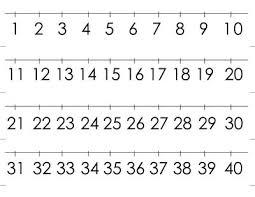Our examples were largely sent or created by pupils and students themselves. Therefore, we would be pleased if you could send us any errors you found, spelling mistakes, or rephasing the example. Thank you!

Leave us a comment of this math problem and its solution (i.e. if it is still somewhat unclear...):Be the first to comment!Tips to related online calculators
Need help calculate sum, simplify or multiply fractions? Try our fraction calculator.
Check out our ratio calculator.
Do you have a linear equation or system of equations and looking for its solution? Or do you have quadratic equation?

## Next similar math problems:

1. New ratio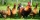The ratio of ducks and chicken in our yard is 2 : 3. The total number of ducks and chickens together is 30. Mother gave 3 of the chickens to our neighbor. What is the new ratio now?
2. Trio ratioHans, Alena and Thomas have a total of 740 USD. Hans and Alena split in the ratio 5: 6 and Alena and Thomas in the ratio 4: 5. How much will everyone get?
3. Gasoline-oil ratioThe manufacturer of a scooter engine recommends a gasoline-oil fuel mixture ratio of 15 to 1. In a particular garage, we can buy pure gasoline and a gasoline-oil mixture, which is 75% gasoline. How much gasoline and how much of the gasoline-oil mix do w
4. PupilsThere are 32 pupils in the classroom, and girls are two-thirds more than boys. a) How many percents are more girls than boys? Round the result to a whole percentage. b) How many are boys in the class? c) Find the ratio of boys and girls in the class. W
5. Two diggersTwo diggers should dig a ditch. If each of them worked just one-third of the time that the other digger needs, they'd dig up a 13/18 ditch together. Find the ratio of the performance of this two diggers.
6. Boys to girlsThe ratio of boys to girls in a party is 3:5 . If 6 more boys arrived and 4 girls left the party, the ratio of boys to girls would be 5:6 . How many are in the party originally?
7. ParkingAt a building parking, 245 spaces are for cars, 56 are for vans and the rest are for buses. If 14% of all parking spaces are for buses, how many parking spaces are there in the building? How many spaces are for buses?Divide number 135 into two additions so that one adds 30 more than 2/5 of the other add. Write the bigger one.
9. Three friendsCuba, Matthew and their friend Adam found a brigade during their weekend weekends because they wanted to make a joint trip to the Alps that they planned for the spring break. Cuba enjoyed the skiing trip very much, so he was not lazy to get up and went
10. The fourThe four pirates divided 65 coins to each other. They were sorted by age, the youngest receiving the least number of coins, each half more than the previous one. How many coins did the oldest pirate receive?Adam has half the money in his right pocket than in his left pocket. If he transferred 40 crowns from the left pocket to the right, he would have the same in both pockets. Calculate how many crowns does Adam have in his left pocket more than in his right?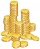The annual scholarship of the best student and second-best student in the class is 3500 euros in total. The best student scholarship in 8 months is the same as the second-best student scholar in the class for the whole year. How big is the annual scholarsThe sum of Robin's age is 45. Seven years ago, Robin was 16 years more than one half as old as Bruno then. How old is Bruno?The cyclist on three-day trip travel 30% of the total route on the first day, 3/5 of the rest on the second day and 35 km on the third day. How many kilometers did travel cyclists each day and how many?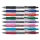Two pen and one notebook costs \$56. One pen costs 1/5 of one notebook. How much is the one notebook?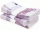The three workers received € 2,850 together for the work done. They divided them according to the time worked so that the first received 20% less than the second and the third € 50 more than the second. How much EUR did each worker receive?The bucket half filled with water weighs 5.55 kg, the full bucket weighs 9.85 kg. How much does the bucket weigh?